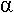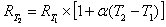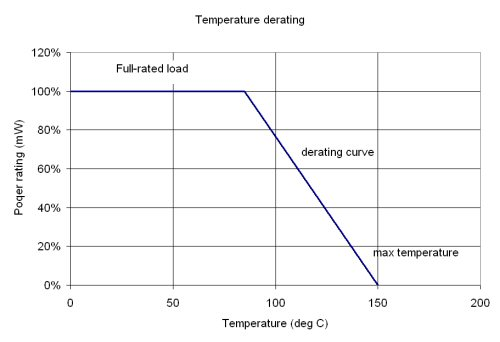# Resistor Mathematics

### Ohm's law

Even at microwave frequencies, resistors obey Ohm's law:

V=IxR

However, in the real world, there is often some non-linearity associated with the term "R". This might be because you are dealing with a semiconductor, which might have a saturation effect, or perhaps because of the temperature effect on resistance due to power dissipation. Watch out!

By the way, one definition of "semiconductor" is a device that doesn't follow Ohm's law. Thus the need for curve tracers!

### Sheet resistance

For April 2010, sheet resistance math has been moved to its own page.

### Skin depth

Skin depth considerations: in a microstrip transmission line, the part of the conductor and ground plane that carry the most current is the closest to the substrate. The skin depth affect is what makes RF sheet resistance greater than DC sheet resistance.

### Resistance temperature coefficient

All resistors exhibit some degree of variation with temperature. Usually the variation is close to linear. The temperature coefficient of resistance,, is usually expressed in ppm/degrees Celsius:The temperature coefficient of resistance can be negative or positive. In the former case the resistance is decreased with temperature. In the latter case it increases with temperature. Pure metals have a positive coefficient. Some alloys have been formulated to have a near-zero temperature coefficient (constantin and maganin for example). Carbon and its associated binders usually has a negative temperature coefficient.

Thermistors are resistors that are built specifically to exploit the temperature coefficient, and are often used as temperature control elements. A thermistor with negative temperature coefficient is called "NTC", while a positive temperature coefficient thermistor is called "PTC".

Did we mention that the temperature coefficient is always at least a slight function of temperature? Something you need to consider, thermistors are not perfectly linear.

### Power derating

Power rating of a resistor specifies the most power that a resistor can dissipate up to a maximum temperature, which will not damage the resistor. Power rating usually implies that a maximum hot-spot temperature must not be reached, surpassing the limit may result in permanent damage

Power rating specifies two temperatures; the first is the temperature up to which the maximum power rating applies, the second temperature is where the rating must be derated to zero dissipation, in between the rating derated linearly with temperature. Two things can be inferred from these ratings: the maximum storage temperature is equal to the derated temperature. Also, the slope of the derating can be used to calculate an equivalent thermal resistance in degrees C per watt. The difference between the no-load and maximum full load temperature is less than or equal to the temperature rise at full load.Let's run through the math, for the component who's derating curve is shown. The calculated thermal resistance is:

Thermal resistance=(150C-85C)/1W=65 degrees C/W

(Thanks for the correction, Rod!)

Nothing could be easier!

Author : Unknown Editor